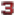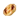> For the similar perk in Zero Hour, see Grenade Belt.

The Ammo Belt is an equipment that appears in Modern Combat 3: Fallen Nation.

## Modern Combat 3: Fallen Nation

{{#infoboxbuilder: | 0:Theme = MC3 default | 0:CustomModule = | 0:MainImageCaption = | 0:ToggleContentLongerThan = 1000

| 1:Type = Title

```| 1:Value = Ammo Belt
```

| 2:Type = MainImage

| 2:Value =

| 3:Type = Line

```| 3:Label = Effect
| 3:Value = Doubles the amount of ammo carried
```

| 4:Type = Line

```| 4:Label = Duration
| 4:Value =
```

| 5:Type = Line

```| 5:Label = Specialization
| 5:Value =
```

| 6:Type = Line

```| 6:Label = Class
| 6:Value =
```

| 7:Type = Line

```| 7:Label = Tier
| 7:Value =
```

| 8:Type = Line

```| 8:Label = Unlocked
| 8:Value = Rank 1
```

| 9:Type = Line

```| 9:Label = Cost
| 9:Value =5,000
```

| 10:Type = Title

```| 10:Value = Ammo Belt Elite
```

| 11:Type = Image

| 11:Value =

| 12:Type = Line

```| 12:Label = Effect
| 12:Value = Triples the amount of ammo carried
```

| 13:Type = Line

```| 13:Label = Unlocked
| 13:Value = Rank 90
```

| 14:Type = Line

```| 14:Label = Cost
| 14:Value =60,000
```

| 15:Type = Title

```| 15:Value =
```

| 16:Type = Image

```| 16:Value =
```

| 17:Type = Line

```| 17:Label = Effect
| 17:Value =
```

| 18:Type = Line

```| 18:Label = Unlocked
| 18:Value =
```

| 19:Type = Line

```| 19:Label = Cost
| 19:Value =

```

| 20:Type = Title

```| 20:Value =
```

| 21:Type = Image

```| 21:Value =
```

| 22:Type = Line

```| 22:Label = Effect
| 22:Value =
```

| 23:Type = Line

```| 23:Label = Unlocked
| 23:Value =
```

| 24:Type = Line

```| 24:Label = Cost
| 24:Value =
```

}}

### Standard Edition

The Standard Edition of the Ammo Belt allows the player to double the amount of ammunition they can carry, so if they carried 150 for an assault rifle, it would be 300.

### Elite Edition

The Elite Edition of the Ammo Belt allows the player to triple the amount of ammuniton they can carry, so carrying 300 for an assault rifle, would add another 150, to a total of 450.